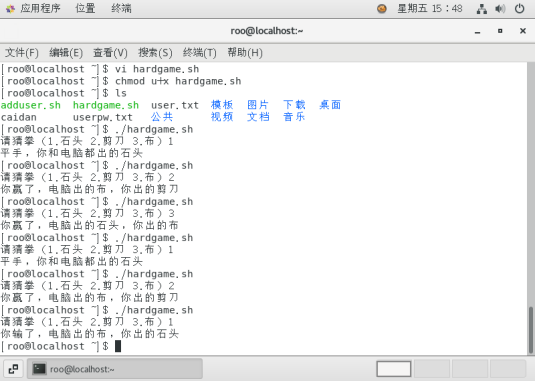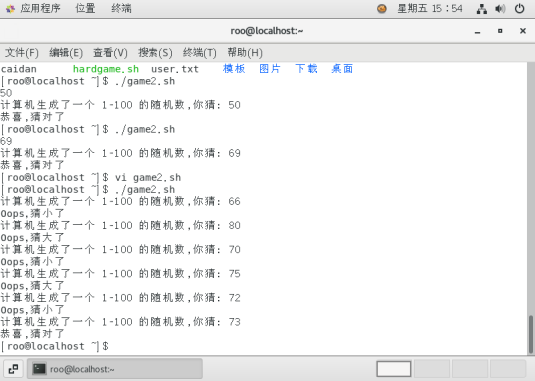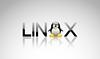• linux操作系统shell编程，源代码，小游戏，俄罗斯方块
• shell小游戏 （1）Shell小游戏–石头剪刀布，结果如下： [root@localhost opt]# ./hardgame.sh 输入1、2、3。1是剪刀，2是石头，3是布:1 电脑出的石头，你赢了 [root@localhost opt]# ./hardgame.sh 输入1、2、3。1...
shell小游戏
（1）Shell小游戏–石头剪刀布，结果如下：
[root@localhost opt]# ./hardgame.sh
输入1、2、3。1是剪刀，2是石头，3是布:1
电脑出的石头，你赢了
[root@localhost opt]# ./hardgame.sh
输入1、2、3。1是剪刀，2是石头，3是布:2
电脑出的石头，打成平手
[root@localhost opt]# ./hardgame.sh
输入1、2、3。1是剪刀，2是石头，3是布:3
电脑出的布，打成平手
源代码:
#!/bin/bash
a=$[RANDOM%3+1] read -p "请猜拳（1.石头 2.剪刀 3.布）" b case "$a""$b" in ) echo "平手，你和电脑都出的石头" ;; ) echo "你输了，电脑出的石头，你出的剪刀" ;; ) echo "你赢了，电脑出的石头，你出的布" ;; ) echo "你赢了，电脑出的剪刀，你出的石头" ;; ) echo "平手，你和电脑都出的剪刀" ;; ) echo "你输了，电脑出的剪刀，你出的布" ;; ) echo "你输了，电脑出的布，你出的石头" ;; ) echo "你赢了，电脑出的布，你出的剪刀" ;; ) echo "平手，你和电脑都出的布" ;; *) echo "请正确出拳" esac  （2）猜数字游戏 #!/bin/bash 脚本生成一个 100 以内的随机数,提示用户猜数字,根据用户的输入,提示用户猜对了, 猜小了或猜大了,直至用户猜对脚本结束。 RANDOM 为系统自带的系统变量,值为 0‐32767的随机数 使用取余算法将随机数变为 1‐100 的随机数 num=$[RANDOM%100+1]
echo “$num” 使用 read 提示用户猜数字 使用 if 判断用户猜数字的大小关系:‐eq(等于),‐ne(不等于),‐gt(大于),‐ge(大于等于), ‐lt(小于),‐le(小于等于) while : do  read -p "计算机生成了一个 1‐100 的随机数,你猜: " cai if [$cai -eq $num ] then echo "恭喜,猜对了" exit elif [$cai -gt $num ] then echo "Oops,猜大了" else echo "Oops,猜小了" fi done  题目就是源代码 源代码： #!/bin/bash # 脚本生成一个 100 以内的随机数,提示用户猜数字,根据用户的输入,提示用户猜对了, # 猜小了或猜大了,直至用户猜对脚本结束。 # RANDOM 为系统自带的系统变量,值为 0‐32767的随机数 # 使用取余算法将随机数变为 1‐100 的随机数 num=$[RANDOM%100+1]
echo "$num" # 使用 read 提示用户猜数字 # 使用 if 判断用户猜数字的大小关系:‐eq(等于),‐ne(不等于),‐gt(大于),‐ge(大于等于), # ‐lt(小于),‐le(小于等于) while : do read -p "计算机生成了一个 1‐100 的随机数,你猜: " cai if [$cai -eq $num ] then echo "恭喜,猜对了" exit elif [$cai -gt $num ] then echo "Oops,猜大了" else echo "Oops,猜小了" fi done  实施效果： (1)石头剪刀布(2)猜数字游戏展开全文bash • 周末，学习的一天，开始学习linux shell，做个笔记。 #!/bin/bash touch ~/test.test for a in$(seq 1 10000000) do echo $a >>test.test done #生成1000层级的目录，最后一级目录中放入文件 for a in ... 周末，学习的一天，开始学习linux shell，做个小笔记。 #!/bin/bash touch ~/test.test for a in$(seq 1 10000000)
do
echo $a >>test.test done #生成1000层级的目录，最后一级目录中放入文件 for a in$(seq 1 1000)
do
echo $a mkdir ./1 #文件夹名称太长会导致文件路径过长而失败 cd ./1 touch$a".test"
if(($a==1000)) then cp ~/test.test ./ fi done  展开全文• SCO、Linux下的shell五子棋小游戏(转)[@more@]偶也尝试用shell编个简单小游戏：代码很笨拙，还不能人机对抗，但必竟能玩了。sco、linux测试通过，linux效果更好些。其它系统未测。代码:#!/bin/...  SCO、Linux下的shell五子棋小游戏(转)[@more@]偶也尝试用shell编个简单小游戏：代码很笨拙，还不能人机对抗，但必竟能玩了。sco、linux测试通过，linux效果更好些。其它系统未测。代码:#!/bin/sh# Programname: five# Author: CLD# 2004.01#L(){# 屏幕任意位置h=$1;l=$2echo -n "[$h;$l;H"}## 界面颜色控制变量white_b="[47;30m" # 白底黑字green_b="[42;30m" # 绿底黑字(移动棋字亮块)blue_b="[44;37m" # 兰底白字gray_b="[40;30;5m" # 灰底黑字gray_w="[40;37;5m" # 灰底白字black_w="[37;40;0m" # 黑底白字# 棋盘换色控制变量brown_b="[43;30m" # 桔黄底黑棋brown_w="[43;37m" # 桔黄底白棋mage_b="[45;30m" # 紫底黑棋mage_w="[45;37m" # 紫底白棋lt_blue_b="[44;30;5m" # 浅兰底黑棋lt_blue_w="[44;37;5m" # 浅兰底白棋kkk(){# 棋盘L 1 22;echo "$gray_b $black_w"L 2 22;echo "$gray_b ┏┯┯┯┯┯┯┯┯┯┯┯┯┯┓ $black_w"L 3 22;echo "$gray_b ┠┼┼┼┼┼┼┼┼┼┼┼┼┼┨ $black_w"L 4 22;echo "$gray_b ┠┼┼┼┼┼┼┼┼┼┼┼┼┼┨ $black_w"L 5 22;echo "$gray_b ┠┼┼╋┼┼┼┼┼┼┼╋┼┼┨ $black_w"L 6 22;echo "$gray_b ┠┼┼┼┼┼┼┼┼┼┼┼┼┼┨ $black_w"L 7 22;echo "$gray_b ┠┼┼┼┼┼┼┼┼┼┼┼┼┼┨ $black_w"L 8 22;echo "$gray_b ┠┼┼┼┼┼┼┼┼┼┼┼┼┼┨ $black_w"L 9 22;echo "$gray_b ┠┼┼┼┼┼┼┼┼┼┼┼┼┼┨ $black_w"L 10 22;echo "$gray_b ┠┼┼┼┼┼┼┼┼┼┼┼┼┼┨ $black_w"L 11 22;echo "$gray_b ┠┼┼┼┼┼┼┼┼┼┼┼┼┼┨ $black_w"L 12 22;echo "$gray_b ┠┼┼┼┼┼┼┼┼┼┼┼┼┼┨ $black_w"L 13 22;echo "$gray_b ┠┼┼╋┼┼┼┼┼┼┼╋┼┼┨ $black_w"L 14 22;echo "$gray_b ┠┼┼┼┼┼┼┼┼┼┼┼┼┼┨ $black_w"L 15 22;echo "$gray_b ┠┼┼┼┼┼┼┼┼┼┼┼┼┼┨ $black_w"L 16 22;echo "$gray_b ┗┷┷┷┷┷┷┷┷┷┷┷┷┷┛ $black_w"L 17 22;echo "$gray_b $black_w"}getchar(){key=stty -gstty -echostty rawdd if=/dev/tty bs=1 count=1 2>/dev/nullstty -rawstty echostty$key}clearkkkL 5 34;echo " 五 子 棋 " L 10 24;echo "┌────游戏○设定────┐"L 11 24;echo "│ │"L 12 24;echo "│ 1_白先走 2_黑先走 [ ] │"L 13 24;echo "│ │"L 14 24;echo "└─────────────┘"L 12 48;echo -ngoo=getcharcase $goo in1) go="白";ss=0;sss=0;;2) go="黑";ss=1;sss=1;;*) clear;exit;;esacby=0;bs=0;bsy=0;hy=0;hs=0;hsy=0 #初始化输赢局数tput civishelp(){L 16 3;echo "┏━━━━━━━┓"L 16 57;echo "┏━━━━━━━┓"L 17 3;echo "┃ ┃"L 17 57;echo "┃ ┃"L 18 3;echo "┃ ┃ ┃ ┃"L 19 3;echo "┃ 赢盘数: ┃┏━━━━━"$gray_b" m---和为贵 "$black_w"━━━━━┓┃ 赢盘数: ┃"L 20 3;echo "┃ 输盘数: ┃┃ 友 比 ┃┃ 输盘数: ┃"L 21 3;echo "┃ ┃┃ 谊 "$white_b"Press c change color"$black_w" 赛 ┃┃ ┃"L 22 3;echo "┃ ┃┃ 第 第 ┃┃ ┃"L 23 3;echo "┃ 最后成绩: ┃┃ 一 二 ┃┃ 最后成绩: ┃"L 24 3;echo "┗━━━━━━━┛┗━━━━━━━━━━━━━━━━┛┗━━━━━━━┛"}init_(){ # 继续棋局初始化kkk >abca1=2 a2=38b1=52b2=16write=$green_b$go$black_wcat abcL $a1$a2;echo $write # 棋子初始位置ccc=1 #改变颜色次数color1=expr substr$gray_b 3 8;color2=expr substr $gray_w 3 8 #默认棋子颜色L 19 13;echo$by;L 19 68;echo $hyL 20 13;echo$bs;L 20 68;echo $hsL 23 15;echo$bsy" ";L 23 70;echo $hsy" "}init_help## 棋子移动函数left(){ ##左移if [$a2 -eq 24 ];then a2=24;else a2=expr $a2 - 2;fisort -t"[" -n +1 abcL$a1 $a2;echo$write}right(){ ##右移if [ $a2 -eq$b1 ];then a2=$b1;else a2=expr$a2 + 2;fisort -t"[" -n +1 abcL $a1$a2;echo $write}down(){ ##下移if [$a1 -eq $b2 ];then a1=$b2;else a1=expr $a1 + 1;fisort -t"[" -n +1 abcL$a1 $a2;echo$write}up(){ ##上移if [ $a1 -eq 2 ];then a1=2;else a1=expr$a1 - 1;fisort -t"[" -n +1 abcL $a1$a2;echo $write}sy(){ # 继续棋局输方先走,统计输赢结果if [$qs -eq 7 ];thengo="黑" #如果白棋赢则下一盘黑先走run="白"bs=expr $bs + 0;by=expr$by + 1bsy=expr $by -$bshy=$bs;hs=$by;hsy=expr $hy -$hselsego="白" #如果黑棋赢则下一盘白先走run="黑"hs=expr $hs + 0;hy=expr$hy + 1hsy=expr $hy -$hsby=$hs;bs=$hy;bsy=expr $by -$bsfi}sy1(){ # 显示输赢结果,统计棋局,询问是否继续 while true;doL 19 13;echo $by;L 19 68;echo$hyL 20 13;echo $bs;L 20 68;echo$hsL 23 15;echo $bsy" ";L 23 70;echo$hsy" "tput bel;tput belL 6 28;echo $white_b" "$black_wL 7 28;echo $white_b" "$run$1"!!! "$black_w" "$black_wL 8 28;echo$white_b" 再来一盘否[y|n]? "$black_w" "$black_wL 9 28;echo $white_b" "$black_w" "$black_wL 10 30;echo " "key=getcharcase$key iny)init_count=expr $count + 1L 23 36;echo "第$count 局"break;;n)clear;cat abcL 7 30;echo $blue_b" "$black_w L 8 30;echo $blue_b" "!!!再见!!!" "$black_w" "$black_wL 9 30;echo$blue_b" "$black_w" "$black_wL 10 30;echo $blue_b" "Author: c1l2d3" "$black_w" "$black_wL 11 30;echo$blue_b" "$black_w" "$black_wL 12 32;echo $black_w" "$black_wtput cnormrm yyy;exit;;*);;esacdone}award() #判断输赢函数{cat abc|sed -n 18,"$"p|awk -F";" '{print$1,$2,$4}'|sed 's/^..//' |awk '{print $1,$2,substr($3,2,1)}' >yyy # 计算输赢临时文件 grep "$qs$" yyy|sort -r -n +0 +1|awk '$2+=(NR*2)' |uniq -c|awk '{print $1}'|grep '[5-9]' >/dev/null 2>&1if [$? -eq 0 ];then sy;sy1 "棋赢啦! ";fi # 横连子>=5 grep "$qs$" yyy|sort -r -n +1|awk '$1+=NR' |uniq -c|awk '{print$1}'|grep '[5-9]' >/dev/null 2>&1if [ $? -eq 0 ];then sy;sy1 "棋赢啦! " ;fi # 竖连子>=5 grep "$qs$" yyy|sort -r -n +0 +1|awk '$1=$1+$2/2'|sort -r -n |awk '$2+=(NR*2)'|uniq -c|awk '{print$1}'|grep '[5-9]' >/dev/null 2>&1if [ $? -eq 0 ];then sy;sy1 "棋赢啦! ";fi # 右斜连子>=5grep "$qs$" yyy|sort -r -n +0 +1|awk '$1=$1-$2/2'|sort -r -n |awk '$2+=(NR*2)'|uniq -c|awk '{print$1}'|grep '[5-9]' >/dev/null 2>&1if [ $? -eq 0 ];then sy;sy1 "棋赢啦! ";fi # 左斜连子>=5}count=1 #局数while truedoL 1 4;echo$white_b" 【执白方】 "$black_wL 4 5;echo$gray_b" a---左移 "$black_wL 6 5;echo$gray_b" d---右移 "$black_wL 8 5;echo$gray_b" w---上移 "$black_wL 10 5;echo$gray_b" s---下移 "$black_wL 12 5;echo$gray_b" p---落子 "$black_wL 14 5;echo$gray_b" ]---投降 "$black_wL 1 62;echo$white_b" 【执黑方】 "$black_wL 4 63;echo$gray_b" 4---左移 "$black_wL 6 63;echo$gray_b" 6---右移 "$black_wL 8 63;echo$gray_b" 8---上移 "$black_wL 10 63;echo$gray_b" 5---下移 "$black_wL 12 63;echo$gray_b" 9---落子 "$black_wL 14 63;echo$gray_b" 0---投降 "$black_wL 22 1;echo -nL 23 36;echo "第$count 局"cc=getcharcase $cc ina|4)if [$ss -eq 0 ] && [ "$cc" = "a" ];then left;fiif [$ss -eq 1 ] && [ "$cc" = "4" ];then left;fi;;d|6)if [$ss -eq 0 ] && [ "$cc" = "d" ];then right;fiif [$ss -eq 1 ] && [ "$cc" = "6" ];then right;fi;;s|5)if [$ss -eq 0 ] && [ "$cc" = "s" ];then down;fiif [$ss -eq 1 ] && [ "$cc" = "5" ];then down;fi;;w|8)if [$ss -eq 0 ] && [ "$cc" = "w" ];then up;fiif [$ss -eq 1 ] && [ "$cc" = "8" ];then up;fi;;m)run="此局和棋! ";sy1;;p|9)if [$ss -eq 0 ] && [ "$cc" = "p" ];thensed -n 18,"$"p abc|grep "[$a1;$a2" 2>&1 if [ $? -eq 0 ];then L 10 32;echo " 此处已有棋...";tput bel;sleep 1write=$green_b白$black_wsss=0elsemm=L$a1 $a2[$color2●$black_wecho$mm>>abcwrite=$green_b黑$black_wsss=1fisort -t"[" -n +1 abcqs=7award;tput bel fiif [ $ss -eq 1 ] && [ "$cc" = "9" ];thensed -n 18,"$"p abc|grep "[$a1;$a2" 2>&1 if [$? -eq 0 ];then L 10 32;echo " 此处已有棋...";tput bel;sleep 1write=$green_b黑$black_wsss=1elsemm=L $a1$a2"[$color1●$black_w"echo $mm>>abcwrite=$green_b白$black_wsss=0fisort -t"[" -n +1 abcqs=0award;tput belfiss=$sss;; # 落子方不允许再走,等待对方走棋0|])if [ "$cc" = "]" ];then go="白";run="白";bs=expr$bs + 1by=expr $by + 0bsy=expr$by - $bshy=$bs;hs=$by;hsy=expr$hy - $hssss=0else go="黑";run="黑";hs=expr$hs + 1;hy=expr $hy + 0hsy=expr$hy - $hsby=$hs;bs=$hy;bsy=expr$by - $bssss=1fiss=$sss #谁投降谁下一局先走sy1 "棋投降啦";;c)md=$ccc # 变换棋盘颜色if [$md -eq 1 ];thendef1=expr substr $gray_b 3 8;def2=expr substr$gray_w 3 8color1=expr substr $brown_b 3 6;color2=expr substr$brown_w 3 6sed 's/'$def1'/'$color1'/g' abc|sed 's/'$def2'/'$color2'/g' >abc.tmpmv abc.tmp abccat abcccc=expr $ccc + 1fi # 桔黄色if [$md -eq 2 ];thendef1=expr substr $brown_b 3 6;def2=expr substr$brown_w 3 6color1=expr substr $lt_blue_b 3 8;color2=expr substr$lt_blue_w 3 8sed 's/'$def1'/'$color1'/g' abc|sed 's/'$def2'/'$color2'/g' >abc.tmpmv abc.tmp abccat abcccc=expr $ccc + 1fi # 浅蓝色if [$md -eq 3 ];thendef1=expr substr $lt_blue_b 3 8;def2=expr substr$lt_blue_w 3 8color1=expr substr $mage_b 3 6;color2=expr substr$mage_w 3 6sed 's/'$def1'/'$color1'/g' abc|sed 's/'$def2'/'$color2'/g' >abc.tmpmv abc.tmp abccat abcccc=expr $ccc - 3fi # 紫色if [$md -eq 0 ];thendef1=expr substr $mage_b 3 6;def2=expr substr$mage_w 3 6color1=expr substr $gray_b 3 8;color2=expr substr$gray_w 3 8sed 's/'$def1'/'$color1'/g' abc|sed 's/'$def2'/'$color2'/g' >abc.tmpmv abc.tmp abccat abcccc=expr $ccc + 1fi # 灰色L 2 38;echo$write;;esacdone图：[myimg]upload/ccc.png[/myimg]

来自 “ ITPUB博客 ” ，链接：http://blog.itpub.net/8225414/viewspace-944888/，如需转载，请注明出处，否则将追究法律责任。

转载于:http://blog.itpub.net/8225414/viewspace-944888/
展开全文• Linux操作系统下，Shell语言编写2048小游戏
• echo "游戏开始" while true do read -p "请b输入一个数: " num2 if [ $num1 -lt$num2 ]; then echo "这个数大了" elif [ $num1 -gt$num2 ]; then echo '这个数了' else echo '输入正确' break fi done done 11...
while true
do
read -p "请a输入一个数：" num1
echo "游戏开始"
while true
do
read -p "请b输入一个数: " num2
if [ $num1 -lt$num2 ];
then
echo "这个数大了"
elif [ $num1 -gt$num2 ];
then
echo '这个数小了'
else
echo '输入正确'
break
fi
done
done
11月7日 12:12




展开全文• 文章目录使用case语句，穷举法运行结果 使用case语句，穷举法 具体代码如下： #!/bin/bash computer=$[$RANDOM%3+1] read -p " 请问你出什么 石头1 剪刀2 布3 ： " player case "$computer$player" in ...运维 服务器
• ## Linux小游戏汇总

千次阅读 2020-04-18 22:34:09
Linux小游戏汇总 1.phear 这是一款GPL协议的小游戏，目标是在不被敌人砸到的时候吃到更多的分数。 示例效果： 界面： 下载指令： $sudo apt-get phear 启动$phear 2.pingus pingus是一款益智解谜游戏，目标是让两...
• Shell使用while do循环制作猜拳小游戏 最近看《Linux就该这么学》这本书想了解一下，在while 条件循环语句章节中看见了一个猜价格小游戏示例，示例如下： Cai.sh #!/bin/bash SUIJISHU=$(expr$RANDOM % 1000) COUNT...
• Linux操作系统下，Shell语言编写2048小游戏 点击下载源码
• 不过read能做的可远不止这些，当从键盘读取用户输入的时候，只有按下回车键才标志输入结束，但是很多时候是没办法按回车键的，输入结束与否是由某个特定字符决定的，例如：在一个游戏当中，当按下+键的时候，小球会...
• 今天就把期间做的一些小游戏源码发上来吧 #!/bin/bash # 菜单 echo "欢迎来到虚空台，凡人，这里你可以得到匹敌世界的财富，拥有灭星的力量，只要...你能活下去" read mainmenu() #返回 {  ...
• 今天就简单来分享一下最近的关于 Linux shell 编程的两个程序。（这里在开头先写个抱歉，程序的代码只有截图） 题目如下： 内容一：猜数游戏 实验步骤： 1） 使用cat命令新建一个shell文件，根据以下提示，构思、...编程实例
• 6、猜数字游戏 #!/bin/bash 脚本生成一个 100 以内的随机数,提示用户猜数字,根据用户的输入,提示用户猜对了, 猜了或猜大了,直至用户猜对脚本结束。 num=$[RANDOM%100+1] RANDOM 为系统自带的系统变量,值为 0‐... • 猜数游戏 执行脚本会随机生成一个1-100之间的数字，由执行者猜，一共有6次机会： 如果在6次...猜数游戏也是初级编程的一个很经典的练习，下面用shell写一下： num=$[ $RANDOM%100+1 ] for ((i=5;i>=0;i--)) do re • 1.一些关于ncurse的知识 （1）使用ncurses原因...//程序退出，调用函数来恢复shell终端的显示，如果没有这句话，shell终端会字乱码，坏掉 keypad();//设置了可以从stdscr接收键盘的功能键（快捷键） //使用keypad（std单链表 链表 c# • 你用别的shell也行，但是bash是很强大的并且也是系统默认的。（学习zsh或tsch只会让你在很多情况下受到限制） 学习 vim 。在Linux下，基本没有什么可与之竞争的编辑器（就算你是一个Emacs或Eclipse的重度用户）... • 改脚本对于shell初学者来说是不错案例选择，仅用619行脚本代码，写出了有趣的小游戏； 环境：Centos7.x 1.dos2unix安装 yum -y install dos2unix 2.格式转换： dos2unix square.sh 3.执行脚本 sh square.sh 备注... • 1.安装Python的运行环境 运行环境就是一系列的...3.猜数字小游戏 代码 import random num = random.randint(1,100) #获取一个随机数 is_done = False #是否猜中的标记 count = 0 #玩家猜了几次 while not is_..python • 文档介绍利用python写"猜数字”，"猜词语”，"...需要在linux中把python3.4编译一下，这样编写python程序时保存为.py格式的文件并添加执行权限再终端运行即可（原理跟shell脚本相同... • 文档介绍利用python写“猜数字”，“猜词语”，“谁是...需要在linux中把python 3.4编译一下，这样编写python程序时保存为.py格式的文件并添加执行权限再终端运行即可（原理跟shell脚本相同），非常方便。ps:贴出来... • shell基础编程题 编写hello world 脚本 通过位置变量创建Linux系统账户及密码 #通过位置变量来创建Linux系统账户及密码 #$1为第一个变量，\$2为第二个变量 猜数字游戏 #脚本生成一个100以内的随机数，提示...
• 而本书除了介绍BASH的知识之外，也有许多有用的关于Linux/UNIX的知识和其他shell的介绍。 在看到本书的英文版后，我决定把它翻译出来，在Linuxsir论坛上结识了译者之一杨春敏共同翻译这本书，600多页的书是本大部头...# linuxshell小游戏linux 订阅